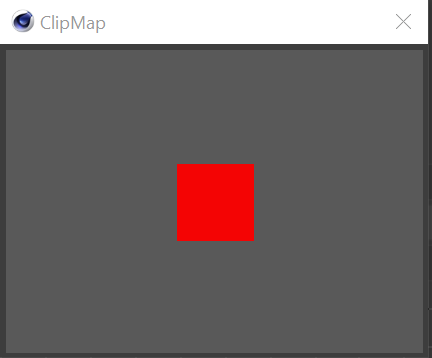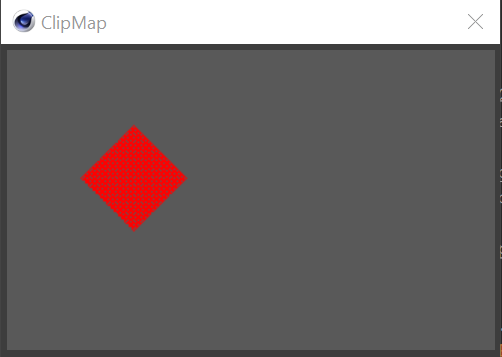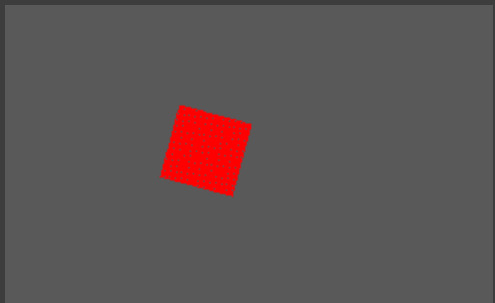# SOLVEDRotating a GeClipMap

Hello,
Is it possible to rotate a GeClipMap? I am drawing a rectangle like so...``````import c4d
from c4d import gui,bitmaps

def drawColorBitmap(w,h,r,g,b):
r = int(r * 255)
g = int(g * 255)
b = int(b * 255)
bmp = bitmaps.BaseBitmap()
bmp.Init(w, h, 24)
for wPixel in range(w):
for hPixel in range(h):
bmp.SetPixel(wPixel, hPixel, r, g, b)
return bmp

class MyUserArea(c4d.gui.GeUserArea):
bitmapWidth = 25
bitmapHeight = 25

def DrawMsg(self, x1, y1, x2, y2, msg):
baseBmp = drawColorBitmap(x2,y2,0.35,0.35,0.35)

drawMap = bitmaps.GeClipMap()
drawMap.InitWithBitmap(baseBmp, None)
drawMap.BeginDraw()
drawMap.SetColor(255,0,0,int(240))
cX = int(self.GetWidth()/2)
cY = int(self.GetHeight()/2)
drawMap.FillRect(int(cX-self.bitmapWidth),
int(cY-self.bitmapHeight),
int(cX+self.bitmapWidth),
int(cY+self.bitmapHeight))
drawMap.SetDrawMode(c4d.GE_CM_DRAWMODE_BLEND,
c4d.GE_CM_SRC_MAX_OPACITY)
drawMap.EndDraw()
bmp = drawMap.GetBitmap()

self.DrawBitmap(bmp,
x1, y1, x2, y2,
0, 0, bmp.GetBw(), bmp.GetBh(),
c4d.BMP_NORMAL | c4d.BMP_ALLOWALPHA)

class ExampleDialog(c4d.gui.GeDialog):
geUserArea = MyUserArea()

def CreateLayout(self):
self.SetTitle("ClipMap")
return True

def main():
dlg = ExampleDialog()
dlg.Open(c4d.DLG_TYPE_MODAL_RESIZEABLE, defaultw=300, defaulth=50)

if __name__=='__main__':
main()
``````

Thank you.

Hi @blastframe,

the short answer to this would be that it is not possible with the Python API, since it does not expose `GeClipMap::FillPolygon` with which it could be done easily in C++. There are also no methods to rotate a `GeClipMap` or a `BaseBitmap` which you could use as an alternative route.

I would also advise against defining a transform yourself like hinted at by @mp5gosu and carried out by you in your code example, since Cinema 4D has a transform type, `c4d.Matrix`, which should be more convenient and faster. So it should look something like this in your case:

``````transformed_point = c4d.Vector(x, y, 0) * c4d.utils.MatrixRotZ(angle_in_radians)
``````

The "grid pattern" you are encountering, is appearing because an antialiased rasterization does not come for free. When you just clamp the final vector components to integer values, you might end up with gaps in some spots (for rotations other than 45°, these patterns will be less regular). Instead a "pixel" that lies between the pixel cells should be split into multiple pixels of different weight. But doing it like you do it at the moment and proposed by @mp5gosu, this would imply some rather elaborate code to properly implement an antialiased rasterization for just some simple box.

Which is why in procedural textures - what is effectively what you are doing here - you usually use signed distance fields (SDF) to represent geometry and then sample that field. The advantage here is that you get the aliasing for free and also can do something like rounded corners or soft edges quite easily. The disadvantage of SDF is that they can exhibit distortions, but that should not be an issue for you here.

About the performance of `GeClipMap.SetPixelRGBA`: It is not in itself especially slow, but the fact that it is usually associated with 10,000's ot 100,000's of calls - to set each pixel - makes it unattractive. If possible you should use a `BaseBitmap` and copy the data in bulk into it. However, doing this 100 times will still be slow, and this is only partially the fault of Python. There is a reason why more flexible GUI-Frameworks like Microsft's WPF run on the GPU, GUI's can be quite taxing if implemented poorly. You should try to cache here as much as possible.

edit: You could also use an external library like `pil` or `pillow` when taking the bitmap route, but I would not take it since you then would have to deliver this external library.

Cheers,
Ferdinand

Easiest would be to define your own Rectangle Class, including a transformation matrix and implement the transformations yourself. This is straight forward and a not so hard task.
This is a common way, since a canvas that is drawn to usually never has a transformation matrix.

http://www.it.hiof.no/~borres/j3d/math/twod/p-twod.html

@mp5gosu Thank you very much for the reply.

I was able to implement the Rectangle class and rotate using sin & cos. My issue now is that there is a strange grid pattern appearing depending on the angle of rotation.

45 degrees165 degreesI thought perhaps it was because of the integers required for the pixel values and the float values coming from the rotation computation, so I tried using `math.floor` and typing to `int` but it didn't fix the issue. This is the draw method for my Rectangle:

``````    def draw(self):
sine=math.sin(self.rotation)
cosine=math.cos(self.rotation)

for wPixel in range(self.width):
for hPixel in range(self.height):
x = wPixel-self.width/2
y = hPixel-self.height/2
new_y=-x*sine+y*cosine
new_x=x*cosine+y*sine
self.clipMap.SetPixelRGBA(int(self.x+new_x), int(self.y+new_y), 255, 0, 0, 255)
``````

Can anyone help me get rid of this strangle pattern?

I also have one concern with this method: @m_magalhaes said SetPixel is really slow in Python. I will have possibly 100 GeClipMaps being rendered every frame.

Hi @blastframe,

the short answer to this would be that it is not possible with the Python API, since it does not expose `GeClipMap::FillPolygon` with which it could be done easily in C++. There are also no methods to rotate a `GeClipMap` or a `BaseBitmap` which you could use as an alternative route.

I would also advise against defining a transform yourself like hinted at by @mp5gosu and carried out by you in your code example, since Cinema 4D has a transform type, `c4d.Matrix`, which should be more convenient and faster. So it should look something like this in your case:

``````transformed_point = c4d.Vector(x, y, 0) * c4d.utils.MatrixRotZ(angle_in_radians)
``````

The "grid pattern" you are encountering, is appearing because an antialiased rasterization does not come for free. When you just clamp the final vector components to integer values, you might end up with gaps in some spots (for rotations other than 45°, these patterns will be less regular). Instead a "pixel" that lies between the pixel cells should be split into multiple pixels of different weight. But doing it like you do it at the moment and proposed by @mp5gosu, this would imply some rather elaborate code to properly implement an antialiased rasterization for just some simple box.

Which is why in procedural textures - what is effectively what you are doing here - you usually use signed distance fields (SDF) to represent geometry and then sample that field. The advantage here is that you get the aliasing for free and also can do something like rounded corners or soft edges quite easily. The disadvantage of SDF is that they can exhibit distortions, but that should not be an issue for you here.

About the performance of `GeClipMap.SetPixelRGBA`: It is not in itself especially slow, but the fact that it is usually associated with 10,000's ot 100,000's of calls - to set each pixel - makes it unattractive. If possible you should use a `BaseBitmap` and copy the data in bulk into it. However, doing this 100 times will still be slow, and this is only partially the fault of Python. There is a reason why more flexible GUI-Frameworks like Microsft's WPF run on the GPU, GUI's can be quite taxing if implemented poorly. You should try to cache here as much as possible.

edit: You could also use an external library like `pil` or `pillow` when taking the bitmap route, but I would not take it since you then would have to deliver this external library.

Cheers,
Ferdinand

@zipit Thank you for explaining this, Ferdinand.

I was able to eliminate the antialiasing by doubling the pixels and scaling the clipmap by 0.5. The performance is slow though (and opacity is inconsistent with the unrotated rectangles) so I think I'm stuck with the aliased version.

Caching
I would like to cache the GeClipMap as a Bitmap to improve performance as you suggested. The reason I'm using GeClipMap is because these rectangles will be rendered with alpha on top of a bitmap (to serve as buttons). Given the example in the initial post, how would I cache this and still keep it interactive?

Mouse Interaction
To determine if the mouse is over a rotated rectangle, I'm using some code that I converted from StackExchange. I'm updating each rectangle's hover state by calling an update method (below). It might be something else, but currently the code seems a bit sluggish. Is there a better way to do this using Cinema 4D's transform?

``````    def update(self,point,scale):
if self.rotation == 0:
if self.x*scale <= point.x <= (self.x+self.width)*scale and self.y*scale <= point.y <= (self.y+self.height)*scale:
self.hover = True
else:
self.hover = False
else:
originX = (self.x + self.width/2)*scale
originY = (self.y + self.height/2)*scale
# translate mouse point values to origin
dx = (point.x - originX)
dy = (point.y - originY)
# distance between the point and the center of the rectangle
h1 = math.sqrt(dx*dx + dy*dy)
currA = math.atan2(dy,dx)
# Angle of point rotated around origin of rectangle in opposition
newA = currA + self.rotation
# New position of mouse point when rotated
x2 = math.cos(newA) * h1
y2 = math.sin(newA) * h1
# Check relative to center of rectangle
if x2 > -0.5 * (self.width*scale) and x2 < 0.5 * (self.width*scale) and y2 > -0.5 * (self.height*scale) and y2 < 0.5 * (self.height*scale):
self.hover = True
else:
self.hover = False
``````

Thanks!

Hi @blastframe,

[...] Is there a better way to do this using Cinema 4D's transform?

The snippet does it how I would also do it, i.e., by rotating the point to test rather trying to hit test a just an arbitrary polygon, this is probably the most important point of optimization. You could also do other things here, by replacing the calculations made for `h1` (`Vector.Length`), `newA` (should be `c4d.utils.GetAngle(localized_mouse_point, self.rotation_as_a_vector)` if I am not overlooking something in your code) and also by using Cinema's matrix type to carry out the calculations made for `x2` and `y2`. But since this is only some hit test code, it should not have a huge impact. Because it is only done once or twice for each frame, opposed to the possible 100,000's calls done for drawing all pixels for each frame. When your code is sluggish, this is probably coming from your draw routine. When unsure who is the culprit, you could profile your `update` method (but from the looks of it your `update` should be below or right at the edge of what can be reliably profiled, because it should be pretty fast).

Given the example in the initial post, how would I cache this and still keep it interactive?

I had not any particular cookie cutter method in mind, because you always have to design such things form case to case. You also did not fully line out how your GUI works, so I'll have to do some guess work here. The general idea is to recompute as little stuff as possible. I will call your rotated boxes "gizmos" and the sum of all gizmos "plate".

1. When the angle and size of your gizmos is static, I would render one - or as many as you need when you have multiple "versions" - into a cache, e.g., some `BaseBitmaps ` or `GeClipMaps`.
2. If even your plate is static, I would instead prerender this into a cache.
3. If your images are being scaled, e.g., a texture file on disk, I would also cache the thumbnails.
4. Since you talk about doing it "100 times", I would assume that you draw outside of the visible area, i.e. you basically create a several megapixel large image and then only display a portion of it in the GUI (the rest is hidden by a scrollbar logic). This should also be avoided, because it will introduce a huge overhead; only draw what you need. If possible, you should cache the whole image, but then make sure it is not being recalculated by just scrolling.
5. Then on a draw call, I would superimpose the images on top of either the  or first build a plate out of  and then superimpose the images.
6. When 5. is also static to some extent, you should also cache it.

There has been recently a similar topic which dealt with points 3. and 4.

Cheers,
Ferdinand

Hi @blastframe,

what I forgot to mention is that when you take the SDF route, you also get the hit-testing for free and more optimized (because these cookie cutter SDF formulas are usually hyper-optimized). A negative distance value for a SDF and an input value, in case of hit testing the localized mouse pointer, will mean that you are inside of the geometry, i.e. it is a hit. A positive distance value will mean that you are outside.

Cheers,
Ferdinand

This post is deleted!

@zipit I just saw your latest message about signed distance fields. I'd be interested in this direction, but have no idea how I'd use the code below for an oriented box in a the GeClipMap (or how to sample it). Is there an example of this in the SDK examples? I searched on the forum for guidance and only found this example for a 3D scene but couldn't derive how to do this in my GUI.

Here is my first attempt at converting signed distance fields code for a rotated box:

``````def sdOrientedBox(p, a, b, th):
l = length(b-a)
d = (b-a)/l
q = (p-(a+b)*0.5)
q = mat2(d.x,-d.y,d.y,d.x)*q #mat2?
q = abs(q)-vec2(l,th)*0.5
return length(max(q,0.0)) + min(max(q.x,q.y),0.0)
``````

For the arguments, I'm guessing p is position (accepting c4d.Vector) and that th is the angle of rotation. I'm unsure of a & b. Perhaps it's the width & height? Also, this function uses a function called `mat2` which I did not understand. I think I'd need to see a working example to go this route.

Hi @blastframe,

you won't find any examples in the SDK. While Cinema's volumes also make use of the term SDF, there are no SDF in the sense of signed distance functions in Cinema's API, the volumes signed distance fields are a related umbrella term but not quite the same. There are however multiple online sources like The Book of Shaders, The Art of Code, Inigo Quilez website and of course the literature which handle that topic extensively. I am as a non-private person are however unfortunately not able to provide you any code examples here, because this topic clearly falls out of the scope of support.

About your question. `mat2` is a `GLSL`, the OpenGL shading language, `2x2` matrix type. But there are also other differences, there is no `length` function in `c4dpy`, you have to use `c4d.Vector.Length` instead, you cannot use `max` like in `GLSL`, you have to evaluate all components of a vector manually, e.g. `max(q.x, q.y, q.z, 0)` and finally there is of course no `vec2`, you have to use `c4d.Vector`. You are also using sort of the wrong formula. You should use the standard two liner for a `sdfBox` and rotate your input coordinates instead, which usually makes things a bit easier than rotating the geometry itself like your function does. Then you have to write a "rasterizer" by iterating over all pixel of your image and querying for each this function. It usually makes things easier when you place your coordinate origin in the middle of the image rather than at the top left. The function will spit out a distance for each pixel, denoting the distance to the shape/surface. If it is positive you are outside, i.e. the color should be bg color/transparent, and if it is negative the color should be the shape's texture. With a `smoothstep` function you can blend the edges of your shape less harshly than with a binary condition like `col = texture if sdf < 0 else background`.

Cheers,
Ferdinand

@zipit Thank you for the information.